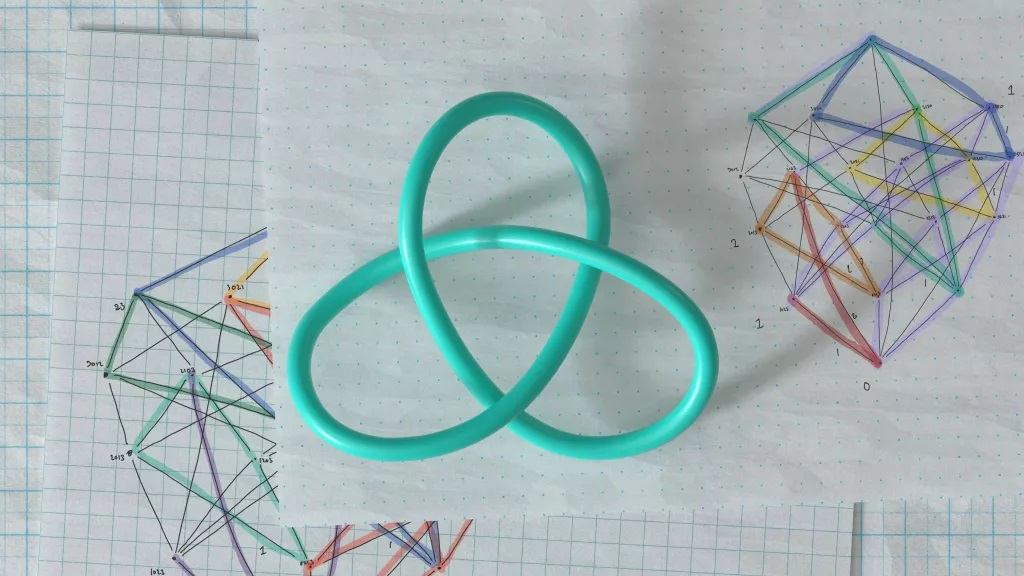# DeepMind cracks ‘knot’ conjecture that bedeviled mathematicians for decadesThe artificial intelligence (AI) program DeepMind has gotten closer to proving a math conjecture that’s bedeviled mathematicians for decades and revealed another new conjecture that may unravel how mathematicians understand knots.

The two pure math conjectures are the first-ever important advances in pure mathematics (or math not directly linked to any non-math application) generated by artificial intelligence, the researchers reported Dec. 1 in the journal Nature. Conjectures are mathematical ideas that are suspected to be true but have yet to be proven in all circumstances. Machine-learning algorithms have previously been used to generate such theoretical ideas in mathematics, but thus far these algorithms have tackled problems smaller than the ones DeepMind has cracked.